# Common Core: 1st Grade Math : Use Rectangles and Circles to Show Halves and Fourths: CCSS.MATH.CONTENT.1.G.A.3

## Example Questions

← Previous 1 3

### Example Question #1 : Use Rectangles And Circles To Show Halves And Fourths: Ccss.Math.Content.1.G.A.3

If I cut this rectangle in half, how many pieces will I have?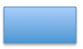Explanation:

When we cut something in half, we getequal pieces.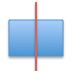### Example Question #2 : Use Rectangles And Circles To Show Halves And Fourths: Ccss.Math.Content.1.G.A.3

If I cut this circle in half, how many pieces will I have?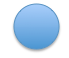Explanation:

When we cut something in half, we get two equal pieces.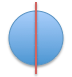### Example Question #84 : Plane Geometry

If I cut this rectangle into fourths, or quarters, how many pieces will I have?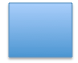Explanation:

When we cut something into fourths, or quarters, we get four equal pieces.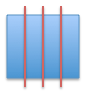### Example Question #84 : Geometry

If I cut this circle into fourths, or quarters, how many pieces will I have?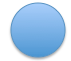Explanation:

When we cut something into fourths, or quarters, we get four equal pieces.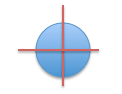### Example Question #2 : Use Rectangles And Circles To Show Halves And Fourths: Ccss.Math.Content.1.G.A.3

If I cut a rectangle into fourths, how am I changing the size?The four pieces will be smaller than the rectangle

The four pieces will be the same size as the rectangle

The four pieces will be different sizes

The four pieces will be bigger than the rectangle

The four pieces will be smaller than the rectangle

Explanation:

When we cut something in fourths, we get four smaller pieces that equal each other. If we put those pieces back together, we’d get the original size of the rectangle.

### Example Question #1 : Use Rectangles And Circles To Show Halves And Fourths: Ccss.Math.Content.1.G.A.3

If I cut a rectangle in half, how am I changing the size?Each piece will be bigger than the rectangle

Each piece will be different sizes

Each pieces will be smaller than the rectangle, they will be half the size.

Each piece will be the same size as the rectangle

Each pieces will be smaller than the rectangle, they will be half the size.

Explanation:

When we cut something in half, we get two smaller pieces that equal each other. If we put those pieces back together, we’d get the original size of the rectangle.

### Example Question #1 : Use Rectangles And Circles To Show Halves And Fourths: Ccss.Math.Content.1.G.A.3

If I cut a rectangle into two parts, I am cutting it in ___________

Fourths

Quarters

Thirds

Half

Half

Explanation:

When we cut something into two parts, which means the same thing as cutting it in half.

### Example Question #2 : Use Rectangles And Circles To Show Halves And Fourths: Ccss.Math.Content.1.G.A.3

What portion of the circle is striped?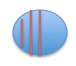A fourth of the circle is stripedd

A fifth of the circle is striped

A third of the circle is striped

Half of the circle is striped

Half of the circle is striped

Explanation:

The circle is divided into two equal sizes. One side is striped and one side is blue. So half of the circle is striped.

### Example Question #3 : Use Rectangles And Circles To Show Halves And Fourths: Ccss.Math.Content.1.G.A.3

A fourth means the same thing as a ____________

Fourth

Third

Quarter

Fifth

Quarter

Explanation:

A fourth and a quarter mean the same thing because both words meanpieces.

### Example Question #4 : Use Rectangles And Circles To Show Halves And Fourths: Ccss.Math.Content.1.G.A.3

If we have two rectangles of the same size, and we cut one of them in half, and one of them in fourths, which pieces will be smaller?The rectangle that we cut into half

They will both have pieces of bigger sizes than the original rectangles

The rectangle that we cut into fourths

They will both have pieces of the same size